Courses

# RD Sharma Solutions -Ex-19.4, Geometrical Constructions, Class 6, Maths Class 6 Notes | EduRev

## RD Sharma Solutions for Class 6 Mathematics

Created by: Abhishek Kapoor

## Class 6 : RD Sharma Solutions -Ex-19.4, Geometrical Constructions, Class 6, Maths Class 6 Notes | EduRev

The document RD Sharma Solutions -Ex-19.4, Geometrical Constructions, Class 6, Maths Class 6 Notes | EduRev is a part of the Class 6 Course RD Sharma Solutions for Class 6 Mathematics.
All you need of Class 6 at this link: Class 6

Q.1 Construct the following angles with the help of protractor :

450, 670, 380, 1100, 1790, 980, 840.

Sol.1 : 450

We draw a ray OA.

We place the protractor on OA such that its center coincides with the point 0 and the diameter of the protractor coincides with OA.

We mark a point B against the mark of 45° on the protractor.

We remove the protractor and draw OB. ∠AOB is the required angle of 45°.

Similarly, we draw the angles 670, 380, 1100, 1790, 980 and 840.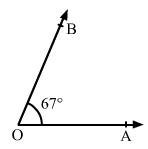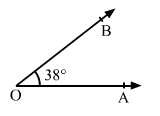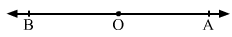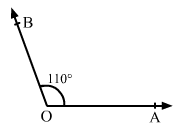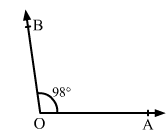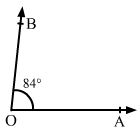Q.2 Draw two rays PQ and RS as shown in figure. Using the protractor, construct angles of 150 and 138with one arm PQ and RS respectively.

Sol.2 : (i) Draw a ray PQ as given in the question.

Place the protractor on the ray PQ such that its center coincides with the point P and the diameter of the protractor coincides with PQ.

Mark a point B against the mark of 15° on the protractor.

Remove the protractor and draw PB. ∠QPB is the required angle of 15°.

(ii) Draw a ray RS as given in the question.

Place the protractor on ray RS such that its centre coincides with the point R and diameter of the protractor coincides with RS.

Mark a point T against the mark of 1380 on the protractor.

Remove the protractor and draw RJ.

∠SRTIIs the required angle of 1380.

103 docs

,

,

,

,

,

,

,

,

,

,

,

,

,

,

,

,

,

,

,

,

,

,

,

,

,

,

,

,

,

,

;